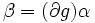# Schur multiplier of finite group is finite and exponent of Schur multiplier divides group order

## Statement

Let$G$ be a finite group. Then the Schur multiplier$M(G)$ of$G$ is finite, and its exponent divides the order of$G$.

## Proof

Let$n$ be the order of$G$.

We show that any 2-cocycle for the action of$G$ on$\mathbb{C}^*$ is cohomologous (viz, a coboundary times) a 2-cocycle with values in the$n^{th}$ roots of unity. To see this let$\alpha$ be a 2-cocycle$\alpha: G \times G \to \mathbb{C}^*$. Then:$\alpha(x,y)\alpha(xy,z) = \alpha(x,yz)\alpha(y,z)$

We now take the product over all$x \in G$. We get:$\prod_{x \in G} \alpha(x,y)\alpha(xy,z) = \prod_{x \in G} \alpha(x,yz) \alpha(y,z)$

Set$t(y) = \prod_{x \in G} \alpha(x,y)$. The above then simplifies to:$t(y)t(z) = t(yz)\alpha(y,z)^n$

For any$x \in G$ choose$g(x) \in \mathbb{C}^*$ such that$g(x)^n = t(x)^{-1}$. Then the coboundary$\partial g$ is the map$(x,y) \mapsto g(xy)/g(x)g(y)$ and we can easily see that$\beta = (\partial g) \alpha$ is a 2-cocycle such that$\beta(x,y)^n = 1$ for all$x,y \in G$. This completes the proof.